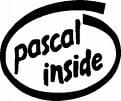Pascal Language - Inverting the elements of an array- recursionHere is a recursive procedure that allows the elements to reverse part of a table between the position p and n:

Procedure Invert (Var t : Tab; p, n : Integer);

Var aux : Real;
Begin
If p < n Then
Begin
aux := t[p];
t[p] := t[n];
t[n] := aux;
Inverser (t, p + 1, n - 1);
End;
End;

Thanks to Zouari Lazhar for this tip